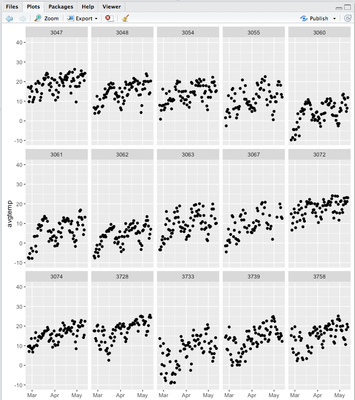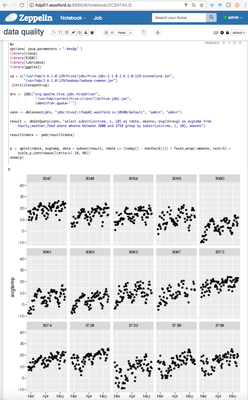Support Questions

## R plots in Zeppelin look fuzzyawoolford
Rising Star

R's ggplot2 is a popular and versatile data visualization package.

The facet plots are a particularly useful method to identify characteristics and anomalies. We can break a scatter plot into facets simply by adding + facet_wrap(~myVar) to an existing plot. Here's an example plot (screenshot from RStudio):Zeppelin supports the R interpreter. Providing R is installed, we can run R commands inside a notebook cell:In this case, we ran a Hive query and created a facet plot.

The question: it looks to me like Zeppelin has created a raster image that's been upscaled and therefore looks fuzzy. In RStudio, the plots look a lot crisper.

I notice that there's an open JIRA for this: https://issues.apache.org/jira/browse/ZEPPELIN-1445

Is there a way to make ggplots look crisp in Zeppelin? Is there a way to render plots as PDF's, i.e. a vector format that doesn't get blurry when scaled, and then display those PDF's inside of the Zeppelin notebook?

1 ACCEPTED SOLUTION

Accepted Solutions

## Re: R plots in Zeppelin look fuzzyawoolford
Rising Star

Zeppelin stores a lot of settings in interpreter.json

The default dpi (dots per inch) for R plot is 72, hence the blurry plots. This value can be increased by adding a dpi property to Zeppelin's R render options. Search for the "zeppelin.R.render.options" key and add "dpi=300":

`"zeppelin.R.render.options": "out.format = 'html', comment = NA, echo = FALSE, results = 'asis', message = F, warning = F, dpi=300",`

You can see an example of the output below:## Re: R plots in Zeppelin look fuzzyawoolford
Rising Star

Zeppelin stores a lot of settings in interpreter.json

The default dpi (dots per inch) for R plot is 72, hence the blurry plots. This value can be increased by adding a dpi property to Zeppelin's R render options. Search for the "zeppelin.R.render.options" key and add "dpi=300":

`"zeppelin.R.render.options": "out.format = 'html', comment = NA, echo = FALSE, results = 'asis', message = F, warning = F, dpi=300",`

You can see an example of the output below:Don't have an account?
Announcements
What's New @ Cloudera
What's New @ Cloudera